# Project 2b: Electromagnetic waves interacting with plasmas of different densities

Imported and adapted from the UCLA Plasma Simulation Group's JupyterPIC Notebooks

Before anything else, we need to change to the stored notebook's directory, which contains input files for the PIC simulator.

cd /notebooks/light-wave-vacuum-into-plasma

In this project you will study what happens when an electromagnetic wave is sent into an unmagnetized plasma. It will illustrate the concepts of cutoffs, reflection, skin depths, and phase and group velocity.

You will simulate plasmas in which each plasma electron is initialized with positions (only in x, or what we call $x_1$) such that the plasma has a nonuniform density profile. In some cases, the density is zero until a specific $x_1=30$, at which time it rises sharply to a value of $n_{max}$. It then remains at this value until $x_1=70$, which is the edge of the simulation window. In other cases, the density is zero until $x_1=10$, at which time is rises to a value of $n_{max}$ between $x_1=10$ and $x_1=50$. It then remains constant until $x_1=70$ (the edge of the simulation window).

The spacing between the electrons is proportional to the inverse of the density. Each electron is also initialized with velocities ($v_1$, $v_2$, $v_3$) = ($.05c$, $.05c$, $.05c$) or momentum ($mv_1$, $mv_2$, $mv_3$) from a Maxwellian in each direction. The particles then begin to move in the self-consistent fields that their current and charge density produce.

At $t=0$, an electromagnetic (EM) wave polarized in the $E_3$ direction with a pulse duration of $\approx 40 \omega_p^{-1}$ is launched by an antenna from the left hand boundary. The peak amplitude is $E_3 = 0.01 mc\omega_p/e$. In the first example, the EM wave has a frequency of $\omega_0 = 0.707$, while in the later examples it has a frequency of $\omega_0 = 1.1$.

## 1. First:

The following lines must always be executed before running anything else:

import osiris as osiris
%matplotlib inline

## 2. Launching Project 2b

In the first cases the density will rise sharply:

• The length of the simulation window is 70 $c/\omega_p$.
• There is no plasma between $x_1=0$ and $x_1=30$.
• For $x_1 > 30$, the density is $n_0$ and the corresponding plasma frequency is $\omega_{p0}$.
• The simulation will run for a time of about 150 $\omega_{p0}^{-1}$.
• The simulation uses 56,000 particles.

In one case $\omega_0 = 0.707\omega_{p0}$ while in a second case $\omega_0 = 1.1\omega_{p0}$.

dirname = 'wall'
osiris.runosiris(rundir=dirname,inputfile='wall.txt')
dirname = 'wall-over'
osiris.runosiris(rundir=dirname,inputfile='wall-over.txt')

In the second group of cases, the density will rise linearly (proportionally to $x_1$):

• The length of the simulation window is 70 $c/\omega_p$.
• There is no plasma between $x_1=0$ and $x_1=10$.
• The plasma rises linearly from $x_1=10$ to $x_1=50$ to a value $n_0$ and the corresponding plasma frequency is $\omega_{p0}$.
• The simulation will run for a time of about 150 $\omega_{p0}^{-1}$.
• The simulation uses 56,000 particles.

In one case $\omega_0 = 0.707\omega_{p0}$ while in a second case $\omega_0 = 1.1\omega_{p0}$.

dirname = 'grad'
osiris.runosiris(rundir=dirname,inputfile='grad.txt')
dirname = 'grad-over'
osiris.runosiris(rundir=dirname,inputfile='grad-over.txt')

## 3. Light wave incident on a very sharp density gradient

dirname = 'wall'
osiris.phasespace(rundir=dirname,dataset='x1',time=0,xlim=[0,70])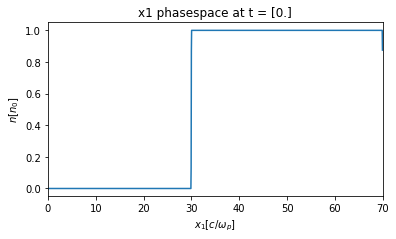Next, you will be plotting $E_3(t, x_1)$, where the color corresponds to the amplitude of the EM wave.

In the first case the density rises sharply and $\omega_0 = 0.707\omega_{p0}$.

dirname = 'wall'
osiris.plot_tx(rundir=dirname, plot_or=3, xlim=[0,70], tlim=[0,150], vmin=-0.01,vmax=0.01, cmap='jet',
show_cutoff=True)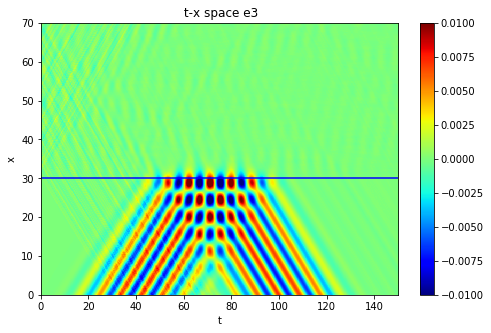• What happened?
• Is the EM wave reflected, absorbed, or transmitted?
dirname = 'wall'
osiris.plot_tx(rundir=dirname, plot_or=3, xlim=[27,33], tlim=[0,150], vmin=-0.01,vmax=0.01, cmap='jet',
show_cutoff=True)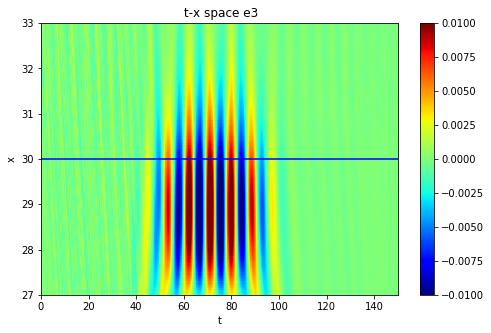• Try to interpert this plot in terms of page 121 in the notes.

## 4. Light wave traveling up a density gradient

Next, the density rises linearly and $\omega_0 = 0.707\omega_{p0}$.

dirname = 'grad'
osiris.phasespace(rundir=dirname,dataset='x1',time=0,xlim=[0,70])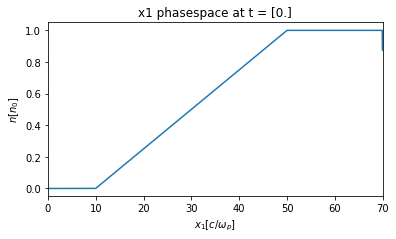dirname = 'grad'
osiris.plot_tx(rundir=dirname, b0_mag=0.0, plot_or=3, xlim=[0,70], tlim=[0,160], vmin=-0.01,vmax=0.01, cmap='jet',
show_cutoff=True)• What looks different in this case?
• Is the EM wave reflected and absorbed?
• At what density does it get absorbed, reflected, or transmitted?
• Can you measure the phase velocity and group velocity where it is reflected?
• Do the phase and group velocity change as the wave moves up the density gradient?
• Is this all consistent with the dispersion relation?

## 5. Light waves with frequency above the electron plasma frequency

For the next two cases $\omega_0 = 1.1\omega_{p0}$.

In the first case, the density rises sharply and $\omega_0 = 1.1\omega_{p0}$.

dirname = 'wall-over'
osiris.phasespace(rundir=dirname,dataset='x1',time=0,xlim=[0,70])dirname = 'wall-over'
osiris.plot_tx(rundir=dirname, plot_or=3, xlim=[0,70], tlim=[0,160], vmin=-0.02,vmax=0.02, cmap='jet',
show_cutoff=True)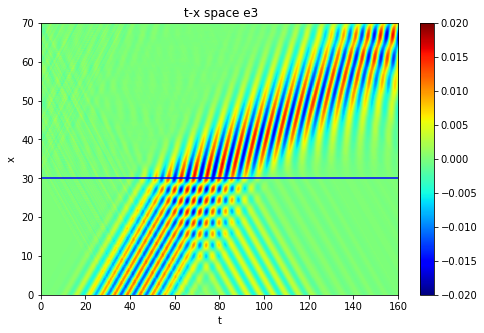• What happened in this case?
• Is the EM wave reflected and/or transmitted?
• How do the phase and group velocity change as the EM wave reaches the peak density?

In the second case the density rises linearly and $\omega_0 = 1.1\omega_{p0}$.

dirname = 'grad-over'
osiris.phasespace(rundir=dirname,dataset='x1',time=0,xlim=[0,70])dirname = 'grad-over'
osiris.plot_tx(rundir=dirname, b0_mag=0.0, plot_or=3, xlim=[0,70], tlim=[0,160], vmin=-0.02,vmax=0.02, cmap='jet',
show_cutoff=True)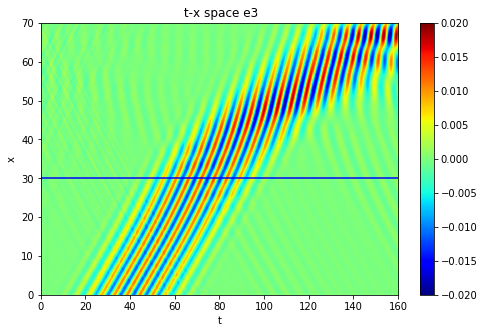• What happened in this case?
• Is the EM wave reflected or transmitted?
• How do the phase and group velocity change as the EM propagates up the density gradient and reaches the peak density?
• Estimate the phase and group velocity at the peak density, does it agree with the dispersion relation?